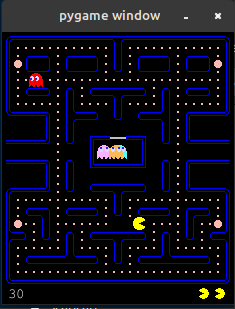# Reinforcement Learning and Pacman agent

Hi everyone, I’d like to create an agent playing pacman based on this paper: Pacman DQN
The pacman that I chose to use is an old project that we made with the university many years ago, the idea of dusting it off seemed nice to me.
The states in the paper are indicated as:

In my case the game screen is a 232x272 matrix resized to 68x68. The states, according to the document, were encoded in a {0,1}-68x68-matrix as followsThe model is as follows:

``````class Deep_QNet(nn.Module):
def __init__(self, num_inputs=7 {or 14?}, num_actions=4):
super().__init__()
self.conv1 = nn.Conv2d(num_inputs, 16, kernel_size=3, stride=1)
self.conv2 = nn.Conv2d(16, 16, kernel_size=3, stride=1)

self.linear1 = nn.Linear(65536, 512)
self.linear2 = nn.Linear(512, num_actions)

def forward(self, x):
#ConvNet
x = F.relu(self.conv1(x))
x = F.relu(self.conv2(x))
x = x.view(x.size(0), -1)
#MLP
x = F.relu(self.linear1(x))
x = self.linear2(x)
return x
``````

I quote the paper:

As a consequence, each frame contains the locations of all game-elements represented in a
W × H × 6 tensor, where W and H are the respective width and height of the game grid.
Conclusively a state is represented by a tuple of two of these tensors together representing the
last two frames, resulting in an input dimension of W × H × 6 × 2.

What confuses me a little, is that later when they illustrate the structure of the model, as input is indicated W × H × 6.
Shouldn’t the network actually accept W × H × 12 input?
(the first 6 are frame 1, the remaining 6 are the following frame)

I have a problem with what input the network should have, taking into account that the long_memory is trained with a batch_size of 32.

My question is therefore the following, the input of the network should be:

• For Short memory: `1×14×68×68` (1 corresponds to the batch_size of short memory) ?
• For Long memory: `32×14×68×68` (32 corresponds to the batch_size of long memory) ?

Could someone clarify how to treat the last two 7-level frames and feed them to the network? Sorry if my ideas are a bit confused.
I hope to be able to run the whole system and create an agent capable of playing pacman.
I would like to share the whole project on github and help those like me who are approaching for the first time.